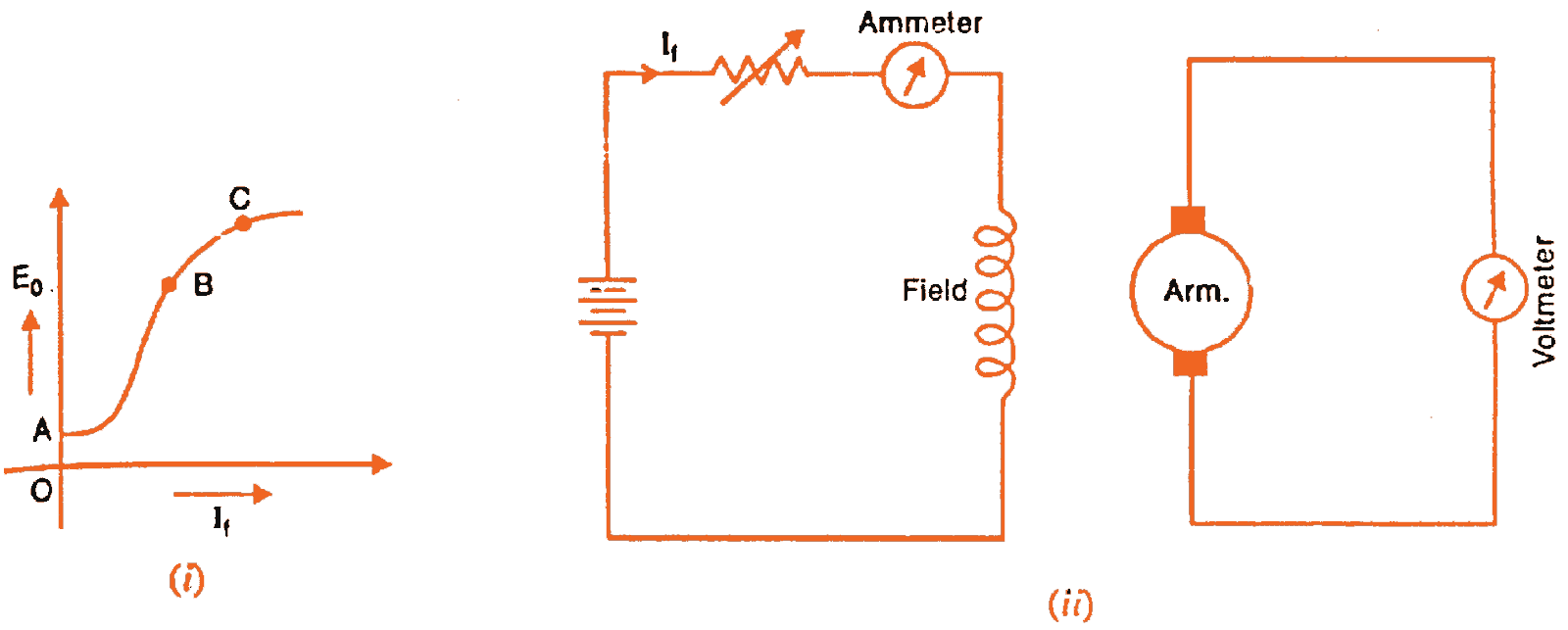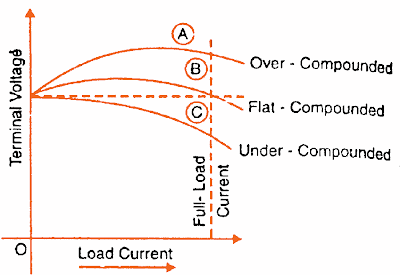# Characteristics Of DC Generators

0
597

## Characteristics Of DC Generators

Generally, following three characteristics of DC generators are taken into considerations:

(i) Open Circuit Characteristic (O.C.C.)

(ii) Internal or Total Characteristic

(iii) External Characteristic

These characteristics of DC generators are explained below.

### 1. Open Circuit Characteristic (O.C.C.) (E/If)

Open circuit characteristic is also known as magnetic characteristic or no-load saturation characteristic. This characteristic shows the relation between generated emf at no load (E) and the field current (If) at a given fixed speed. The O.C.C. curve is just the magnetization curve and it is practically similar for all type of generators. The data for O.C.C. curve is obtained by operating the generator at no load and keeping a constant speed. Field current is gradually increased and the corresponding terminal voltage is recorded. The connection arrangement to obtain O.C.C. curve is as shown in the figure below. For shunt or series excited generators, the field winding is disconnected from the machine and connected across an external supply.Now, from the emf equation of dc generator, we know that Eg = kɸ. Hence, the generated emf should be directly proportional to field flux (and hence, also directly proportional to the field current). However, even when the field current is zero, some amount of emf is generated (represented by OA in the figure below). This initially induced emf is due to the fact that there exists some residual magnetism in the field poles. Due to the residual magnetism, a small initial emf is induced in the armature. This initially induced emf aids the existing residual flux, and hence, increasing the overall field flux. This consequently increases the induced emf. Thus, O.C.C. follows a straight line. However, as the flux density increases, the poles get saturated and the ɸ becomes practically constant. Thus, even we increase the If further, ɸ remains constant and hence, Eg also remains constant. Hence, the O.C.C. curve looks like the B-H characteristic.

READ HERE  Armature Reaction In DC MachinesThe above figure shows a typical no-load saturation curve or open circuit characteristics for all types of DC generators.

### 2. Internal Or Total Characteristic (E/Ia)

An internal characteristic curve shows the relation between the on-load generated emf (Eg) and the armature current (Ia). The on-load generated emf Eg is always less than E due to the armature reaction. Eg can be determined by subtracting the drop due to demagnetizing effect of armature reaction from no-load voltage E. Therefore, internal characteristic curve lies below the O.C.C. curve.

### 3. External Characteristic (V/IL)

An external characteristic curve shows the relation between terminal voltage (V) and the load current (IL). Terminal voltage V is less than the generated emf Eg due to voltage drop in the armature circuit. Therefore, external characteristic curve lies below the internal characteristic curve. External characteristics are very important to determine the suitability of a generator for a given purpose. Therefore, this type of characteristic is sometimes also called as performance characteristic or load characteristic.

Internal and external characteristic curves are shown below for each type of generator.

## Characteristics Of Separately Excited DC GeneratorIf there is no armature reaction and armature voltage drop, the voltage will remain constant for any load current. Thus, the straight line AB in above figure represents the no-load voltage vs. load current IL. Due to the demagnetizing effect of armature reaction, the on-load generated emf is less than the no-load voltage. The curve AC represents the on-load generated emf Eg vs. load current IL i.e. internal characteristic (as Ia = IL for a separately excited dc generator). Also, the terminal voltage is lesser due to ohmic drop occurring in the armature and brushes. The curve AD represents the terminal voltage vs. load current i.e. external characteristic.

READ HERE  Brushless DC Motor (BLDC) – Construction, Working & Applications

## Characteristics Of DC Shunt Generator

To determine the internal and external load characteristics of a DC shunt generator the machine is allowed to build up its voltage before applying any external load. To build up voltage of a shunt generator, the generator is driven at the rated speed by a prime mover. Initial voltage is induced due to residual magnetism in the field poles. The generator builds up its voltage as explained by the O.C.C. curve. When the generator has built up the voltage, it is gradually loaded with resistive load and readings are taken at suitable intervals. Connection arrangement is as shown in the figure below.Unlike, separately excited DC generator, here, IL≠Ia. For a shunt generator, Ia=IL+If. Hence, the internal characteristic can be easily transmitted to Eg vs. IL by subtracting the correct value of If from Ia.During a normal running condition, when load resistance is decreased, the load current increases. But, as we go on decreasing the load resistance, terminal voltage also falls. So, load resistance can be decreased up to a certain limit, after which the terminal voltage drastically decreases due to excessive armature reaction at very high armature current and increased I2R losses. Hence, beyond this limit any further decrease in load resistance results in decreasing load current. Consequently, the external characteristic curve turns back as shown by dotted line in the above figure.

READ HERE  Losses In A DC Generator And DC Motor

## Characteristics Of DC Series GeneratorThe curve AB in above figure identical to open circuit characteristic (O.C.C.) curve. This is because in DC series generators field winding is connected in series with armature and load. Hence, here load current is similar to field current (i.e. IL=If). The curve OC and OD represent internal and external characteristic respectively. In a DC series generator, terminal voltage increases with the load current. This is because, as the load current increases, field current also increases. However, beyond a certain limit, terminal voltage starts decreasing with increase in load. This is due to excessive demagnetizing effects of the armature reaction.

## Characteristics Of DC Compound GeneratorThe above figure shows the external characteristics of DC compound generators. If series winding amp-turns are adjusted so that, increase in load current causes increase in terminal voltage then the generator is called to be over compounded. The external characteristic for over compounded generator is shown by the curve AB in above figure.
If series winding amp-turns are adjusted so that, the terminal voltage remains constant even the load current is increased, then the generator is called to be flat compounded. The external characteristic for a flat compounded generator is shown by the curve AC.
If the series winding has lesser number of turns than that would be required to be flat compounded, then the generator is called to be under compounded. The external characteristics for an under compounded generator are shown by the curve AD.